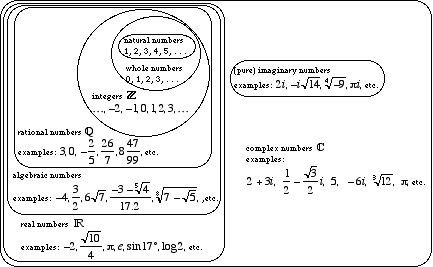index: click on a letter A B C D E F G H I J K L M N O P Q R S T U V W X Y Z A to Z index index: subject areas numbers & symbols sets, logic, proofs geometry algebra trigonometry advanced algebra & pre-calculus calculus advanced topics probability & statistics real world applications multimedia entrieswww.mathwords.com about mathwords website feedback

 Transcendental Numbers Real numbers that are not algebraic. That is, real numbers that cannot be a root of a polynomial equation with integer coefficients. e and π are transcendental.See also